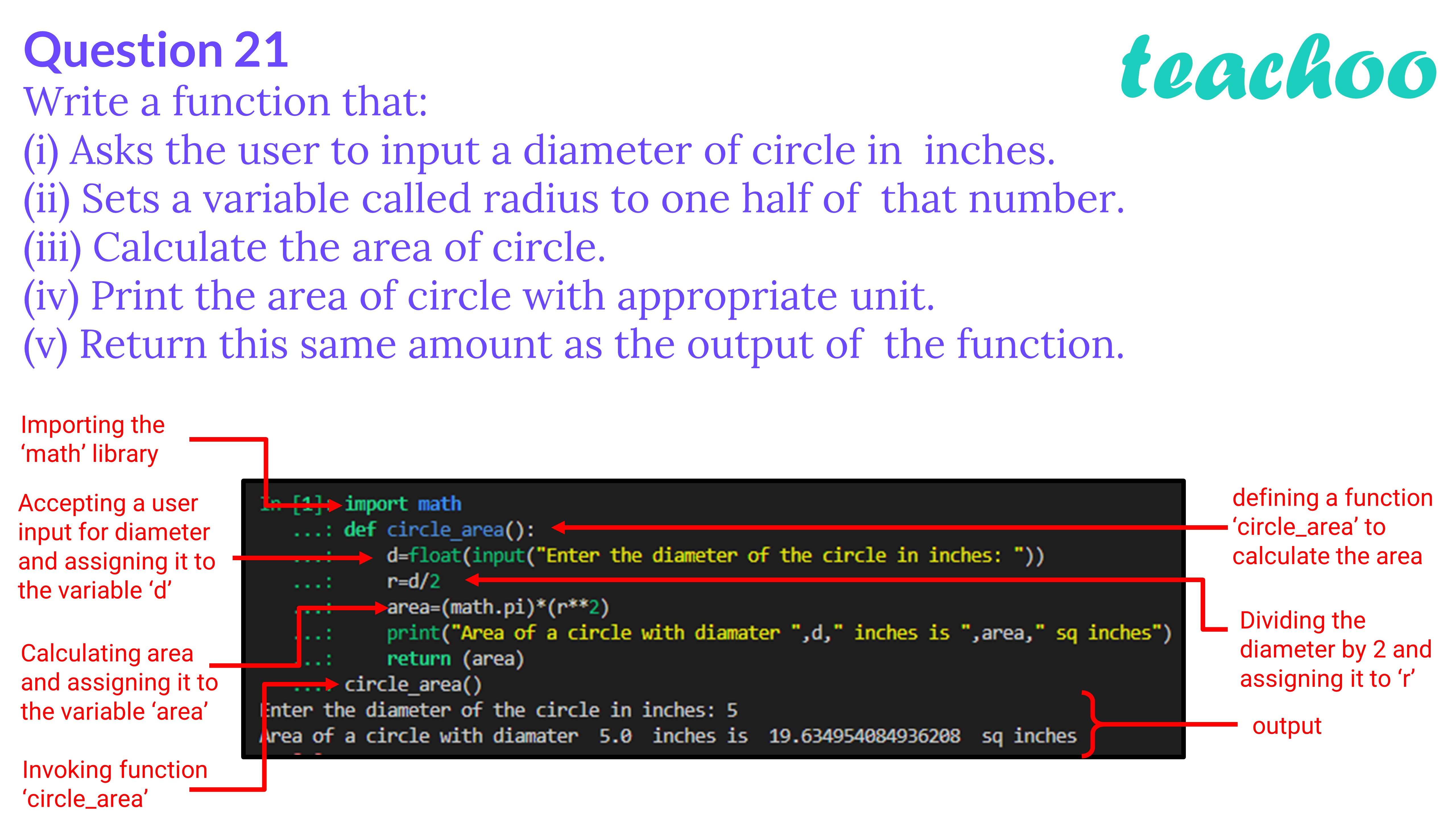Past Year - 2 Mark Questions

Computer Science - Class 12
Chapter 14 - Functions in python

## (v) Return this same amount as the output of the function.

``` import math ```

``` def circle_area (): ```

```     d=float(input( "Enter the diameter of the circle in inches: " )) ```

```     r=d/ 2 ```

```     area=(math.pi)*(r** 2 ) ```

```     print( "Area of a circle with diameter " ,d, " inches is " ,area, " sq inches" ) ```

```     return (area) ```Learn in your speed, with individual attention - Teachoo Maths 1-on-1 Class

### Transcript

Question 21 Write a function that: (i) Asks the user to input a diameter of circle in inches. (ii) Sets a variable called radius to one half of that number. (iii) Calculate the area of circle. (iv) Print the area of circle with appropriate unit. (v) Return this same amount as the output of the function. Importing the ‘math’ library Accepting a user input for diameter and assigning it to the variable ‘d’ Calculating area and assigning it to the variable ‘area’ Invoking function ‘circle_area’ defining a function ‘circle_area’ to calculate the area Dividing the diameter by 2 and assigning it to ‘r’ output